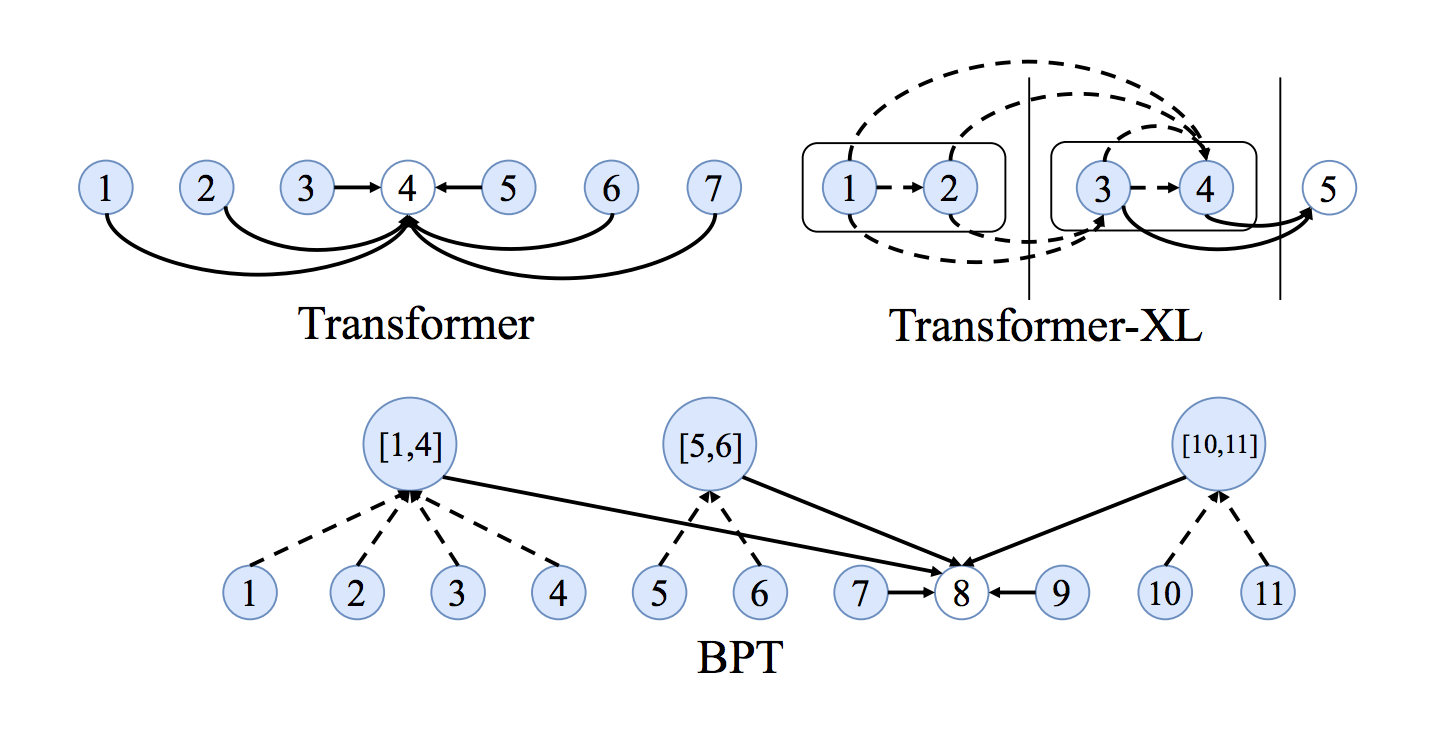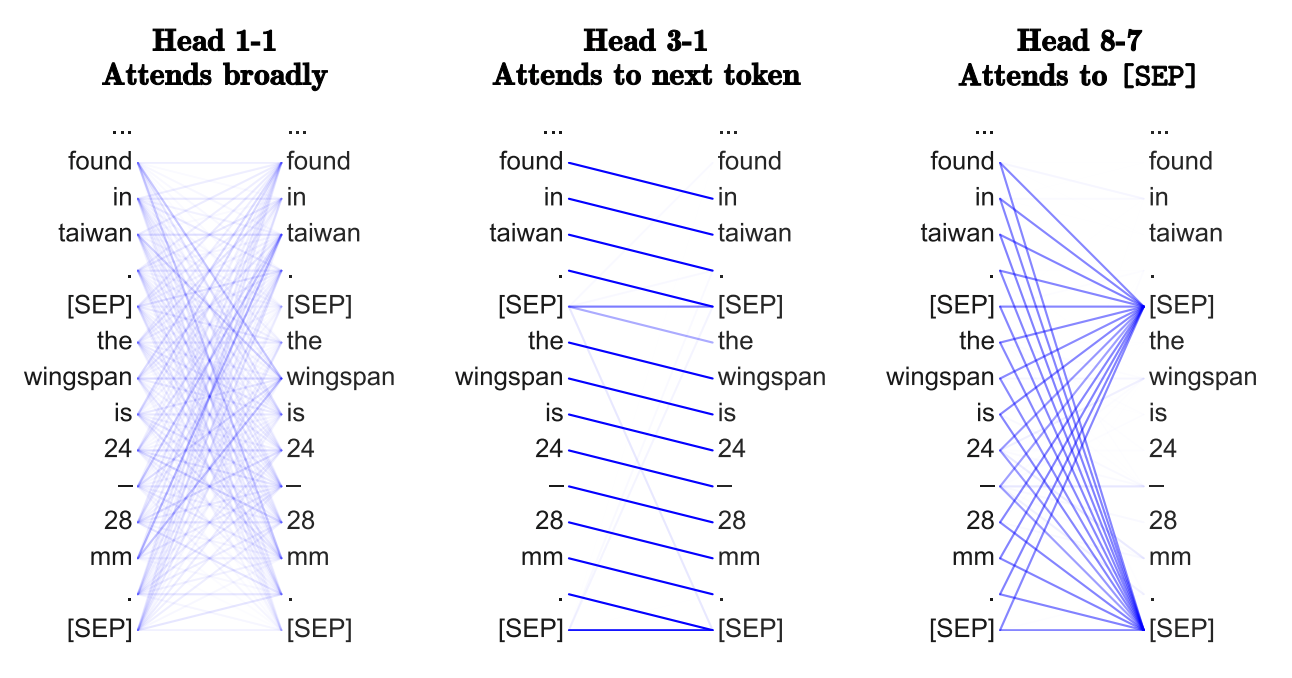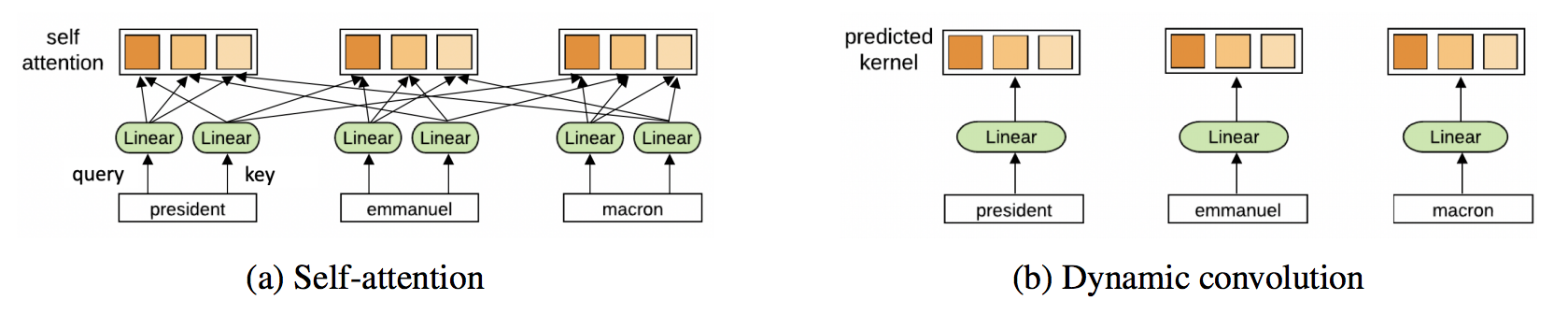# Transformers are Graph Neural Networks

My engineering friends often ask me: deep learning on graphs sounds great, but are there any real applications?

While Graph Neural Networks are used in recommendation systems at Pinterest, Alibaba and Twitter, a more subtle success story is the Transformer architecture, which has taken the NLP world by storm. Through this post, I want to establish a link between Graph Neural Networks (GNNs) and Transformers. I'll talk about the intuitions behind model architectures in the NLP and GNN communities, make connections using equations and figures, and discuss how we can work together to drive future progress. Let's start by talking about the purpose of model architectures—representation learning.

### Representation Learning for NLP

At a high level, all neural network architectures build representations of input data as vectors/embeddings, which encode useful statistical and semantic information about the data. These latent or hidden representations can then be used for performing something useful, such as classifying an image or translating a sentence. The neural network learns to build better-and-better representations by receiving feedback, usually via error/loss functions.

For Natural Language Processing (NLP), conventionally, Recurrent Neural Networks (RNNs) build representations of each word in a sentence in a sequential manner, i.e., one word at a time. Intuitively, we can imagine an RNN layer as a conveyor belt, with the words being processed on it autoregressively from left to right. In the end, we get a hidden feature for each word in the sentence, which we pass to the next RNN layer or use for our NLP tasks of choice.

I highly recommend Chris Olah's legendary blog for recaps on RNNs and representation learning for NLP.

Initially introduced for machine translation, Transformers have gradually replaced RNNs in mainstream NLP. The architecture takes a fresh approach to representation learning: Doing away with recurrence entirely, Transformers build features of each word using an attention mechanism to figure out how important all the other words in the sentence are w.r.t. to the aforementioned word. Knowing this, the word's updated features are simply the sum of linear transformations of the features of all the words, weighted by their importance.

Back in 2017, this idea sounded very radical, because the NLP community was so used to the sequential—one-word-at-a-time—style of processing text with RNNs. The title of the paper probably added fuel to the fire! For a recap, Yannic Kilcher made an excellent video overview.

### Breaking down the Transformer

Let's develop intuitions about the architecture by translating the previous paragraph into the language of mathematical symbols and vectors.
We update the hidden feature $h$ of the $i$'th word in a sentence $\mathcal{S}$ from layer $\ell$ to layer $\ell+1$ as follows:

$$h_{i}^{\ell+1} = \text{Attention} \left( Q^{\ell} h_{i}^{\ell} \ , K^{\ell} h_{j}^{\ell} \ , V^{\ell} h_{j}^{\ell} \right)$$
i.e., $$\ h_{i}^{\ell+1} = \sum_{j \in \mathcal{S}} w_{ij} \left( V^{\ell} h_{j}^{\ell} \right)$$

$$\text{where} \ w_{ij} = \text{softmax}_j \left ( Q^{\ell} h^{\ell}_i \cdot K^{\ell} h^{\ell}_j \right)$$

where $j \in \mathcal{S}$ denotes the set of words in the sentence and $Q^{\ell}, K^{\ell}, V^{\ell}$ are learnable linear weights (denoting the Query, Key and Value for the attention computation, respectively).
The attention mechanism is performed parallelly for each word in the sentence to obtain their updated features in one shot—another plus point for Transformers over RNNs, which update features word-by-word.

We can understand the attention mechanism better through the following pipeline:Taking in the features of the word $h_{i}^{\ell}$ and the set of other words in the sentence $\{ h_{j}^{\ell} \;\ \forall j \in \mathcal{S} \}$, we compute the attention weights $w_{ij}$ for each pair $(i,j)$ through the dot-product, followed by a softmax across all $j$ 's. Finally, we produce the updated word feature $h_{i}^{\ell+1}$ for word $i$ by summing over all $\{ h_{j}^{\ell} \}$ 's weighted by their corresponding $w_{ij}$. Each word in the sentence parallelly undergoes the same pipeline to update its features.

### Multi-head Attention

Getting this straightforward dot-product attention mechanism to work proves to be tricky. Bad random initializations of the learnable weights can de-stabilize the training process.
We can overcome this by parallelly performing multiple 'heads' of attention and concatenating the result (with each head now having separate learnable weights):

$$h_{i}^{\ell+1} = \text{Concat} \left( \text{head}_1, \ldots, \text{head}_K \right) O^{\ell},$$

$$\text{head}_k = \text{Attention} \left( Q^{k,\ell} h_i^{\ell} \ , K^{k, \ell} h_j^{\ell} \ , V^{k, \ell} h_j^{\ell} \right),$$

where $Q^{k,\ell}, K^{k,\ell}, V^{k,\ell}$ are the learnable weights of the $k$'th attention head and $O^{\ell}$ is a down-projection to match the dimensions of $h_i^{\ell+1}$ and $h_i^{\ell}$ across layers.

Multiple heads allow the attention mechanism to essentially 'hedge its bets', looking at different transformations or aspects of the hidden features from the previous layer. We'll talk more about this later.

### Scaling Issues

A key issue motivating the final Transformer architecture is that the features for words after the attention mechanism might be at different scales or magnitudes. This can be due to some words having very sharp or very distributed attention weights $w_{ij}$ when summing over the features of the other words. Additionally, at the individual feature/vector entries level, concatenating across multiple attention heads—each of which might output values at different scales—can lead to the entries of the final vector $h_{i}^{\ell+1}$ having a wide range of values.
Following conventional ML wisdom, it seems reasonable to add a normalization layer into the pipeline.

Transformers overcome issue (2) with LayerNorm, which normalizes and learns an affine transformation at the feature level. Additionally, scaling the dot-product attention by the square-root of the feature dimension helps counteract issue (1).

Finally, the authors propose another 'trick' to control the scale issue: a position-wise 2-layer MLP with a special structure. After the multi-head attention, they project $h_i^{\ell+1}$ to a (absurdly) higher dimension by a learnable weight, where it undergoes the ReLU non-linearity, and is then projected back to its original dimension followed by another normalization:

$$h_i^{\ell+1} = \text{LN} \left( \text{MLP} \left( \text{LN} \left( h_i^{\ell+1} \right) \right) \right)$$

To be honest, I'm not sure what the exact intuition behind the over-parameterized feed-forward sub-layer was. I suppose LayerNorm and scaled dot-products didn't completely solve the issues highlighted, so the big MLP is a sort of hack to re-scale the feature vectors independently of each other. According to Jannes Muenchmeyer, the feed-forward sub-layer ensures that the Transformer is a universal approximator. Thus, projecting to a very high dimensional space, applying a non-linearity, and re-projecting to the original dimension allows the model to represent more functions than maintaining the same dimension across the hidden layer would.

The final picture of a Transformer layer looks like this:

The Transformer architecture is also extremely amenable to very deep networks, enabling the NLP community to scale up in terms of both model parameters and, by extension, data.
Residual connections between the inputs and outputs of each multi-head attention sub-layer and the feed-forward sub-layer are key for stacking Transformer layers (but omitted from the diagram for clarity).

### GNNs build representations of graphs

Let's take a step away from NLP for a moment.

Graph Neural Networks (GNNs) or Graph Convolutional Networks (GCNs) build representations of nodes and edges in graph data. They do so through neighbourhood aggregation (or message passing), where each node gathers features from its neighbours to update its representation of the local graph structure around it. Stacking several GNN layers enables the model to propagate each node's features over the entire graph—from its neighbours to the neighbours' neighbours, and so on.Take the example of this emoji social network: The node features produced by the GNN can be used for predictive tasks such as identifying the most influential members or proposing potential connections.

In their most basic form, GNNs update the hidden features $h$ of node $i$ (for example, 😆) at layer $\ell$ via a non-linear transformation of the node's own features $h_i^{\ell}$ added to the aggregation of features $h_j^{\ell}$ from each neighbouring node $j \in \mathcal{N}(i)$:

$$h_{i}^{\ell+1} = \sigma \Big( U^{\ell} h_{i}^{\ell} + \sum_{j \in \mathcal{N}(i)} \left( V^{\ell} h_{j}^{\ell} \right) \Big),$$

where $U^{\ell}, V^{\ell}$ are learnable weight matrices of the GNN layer and $\sigma$ is a non-linear function such as ReLU. In the example, $\mathcal{N}$(😆) $=$ { 😘, 😎, 😜, 🤩 }.

The summation over the neighbourhood nodes $j \in \mathcal{N}(i)$ can be replaced by other input size-invariant aggregation functions such as simple mean/max or something more powerful, such as a weighted sum via an attention mechanism.

Does that sound familiar? Maybe a pipeline will help make the connection:

If we were to do multiple parallel heads of neighbourhood aggregation and replace summation over the neighbours $j$ with the attention mechanism, i.e., a weighted sum, we'd get the Graph Attention Network (GAT). Add normalization and the feed-forward MLP, and voila, we have a Graph Transformer!

### Sentences are fully-connected word graphs

To make the connection more explicit, consider a sentence as a fully-connected graph, where each word is connected to every other word. Now, we can use a GNN to build features for each node (word) in the graph (sentence), which we can then perform NLP tasks with.

Broadly, this is what Transformers are doing: they are GNNs with multi-head attention as the neighbourhood aggregation function. Whereas standard GNNs aggregate features from their local neighbourhood nodes $j \in \mathcal{N}(i)$, Transformers for NLP treat the entire sentence $\mathcal{S}$ as the local neighbourhood, aggregating features from each word $j \in \mathcal{S}$ at each layer.

Importantly, various problem-specific tricks—such as position encodings, causal/masked aggregation, learning rate schedules and extensive pre-training—are essential for the success of Transformers but seldom seem in the GNN community. At the same time, looking at Transformers from a GNN perspective could inspire us to get rid of a lot of the bells and whistles in the architecture.

# Lessons

### Are sentences fully connected graphs?

Now that we've established a connection between Transformers and GNNs, let me throw some ideas around. For one, are fully-connected graphs the best input format for NLP?

Before statistical NLP and ML, linguists like Noam Chomsky focused on developing formal theories of linguistic structure, such as syntax trees/graphs. Tree LSTMs already tried this, but maybe Transformers/GNNs are better architectures for bringing together the two worlds of linguistic theory and statistical NLP? For example, a very recent work from MILA and Stanford explores augmenting pre-trained Transformers such as BERT with syntax trees [Sachan et al., 2020].Source: Wikipedia

### Long term dependencies

Another issue with fully-connected graphs is that they make learning very long-term dependencies between words difficult. This is simply due to how the number of edges in the graph scales quadratically with the number of nodes, i.e., in an $n$ word sentence, a Transformer/GNN would be doing computations over $n^2$ pairs of words. Things get out of hand for very large $n$.

The NLP community's perspective on the long sequences and dependencies problem is interesting: making the attention mechanism sparse or adaptive in terms of input size, adding recurrence or compression into each layer, and using Locality Sensitive Hashing for efficient attention are all promising new ideas for better transformers. See Maddison May's excellent survey on long-term context in Transformers for more details.

It would be interesting to see ideas from the GNN community thrown into the mix, e.g., Binary Partitioning for sentence graph sparsification seems like another exciting approach. BP-Transformers recursively sub-divide sentences into two until they can construct a hierarchical binary tree from the sentence tokens. This structural inductive bias helps the model process longer text sequences in a memory-efficient manner.Source: Ye et al., 2019

### Are Transformers learning neural syntax?

There have been several interesting papers from the NLP community on what Transformers might be learning. The basic premise is that performing attention on all word pairs in a sentence—with the purpose of identifying which pairs are the most interesting—enables Transformers to learn something like a task-specific syntax. Different heads in the multi-head attention might also be 'looking' at different syntactic properties.

In graph terms, by using GNNs on full graphs, can we recover the most important edges—and what they might entail—from how the GNN performs neighborhood aggregation at each layer? I'm not so convinced by this view yet.Source: Clark et al., 2019

### Why multiple heads of attention? Why attention?

I'm more sympathetic to the optimization view of the multi-head mechanism—having multiple attention heads improves learning and overcomes bad random initializations. For instance, these papers showed that Transformer heads can be 'pruned' or removed after training without significant performance impact.

Multi-head neighbourhood aggregation mechanisms have also proven effective in GNNs, e.g., GAT uses the same multi-head attention, and MoNet uses multiple Gaussian kernels for aggregating features. Although invented to stabilize attention mechanisms, could the multi-head trick become standard for squeezing out extra model performance?

Conversely, GNNs with simpler aggregation functions such as sum or max do not require multiple aggregation heads for stable training. Wouldn't it be nice for Transformers if we didn't have to compute pair-wise compatibilities between each word pair in the sentence?

Could Transformers benefit from ditching attention, altogether? Yann Dauphin and collaborators' recent work suggests an alternative ConvNet architecture. Transformers, too, might ultimately be doing something similar to ConvNets!Source: Wu et al., 2019

### Why is training Transformers so hard?

Reading new Transformer papers makes me feel that training these models requires something akin to black magic when determining the best learning rate schedule, warmup strategy and decay settings. This could simply be because the models are so huge and the NLP tasks studied are so challenging.

But recent results suggest that it could also be due to the specific permutation of normalization and residual connections within the architecture.

I know I'm ranting, but this makes me skeptical: Do we really need multiple heads of expensive pair-wise attention, overparameterized MLP sub-layers, and complicated learning schedules? Do we really need massive models with massive carbon footprints? Shouldn't architectures with good inductive biases for the task at hand be easier to train?

## Further Reading

To dive deep into the Transformer architecture from an NLP perspective, check out these amazing blog posts: The Illustrated Transformer and The Annotated Transformer.

Also, this blog isn't the first to link GNNs and Transformers. Here's an excellent talk by Arthur Szlam on the history and connection between Attention/Memory Networks, GNNs and Transformers. Similarly, DeepMind's star-studded position paper introduces the Graph Networks framework, unifying all these ideas. For a code walkthrough, the DGL team has a nice tutorial on seq2seq as a graph problem and building Transformers as GNNs.

### Final Notes

The post initially appeared on the NTU Graph Deep Learning lab website and Medium, and has also been translated to Chinese and Russian. Do join the discussion on Twitter, Reddit or HackerNews!

Author Bio

Chaitanya K. Joshi is a Research Engineer at A*STAR, Singapore, working on Graph Neural Networks and their applications to accelerating scientific discovery. He obtained a BEng in Computer Science from NTU, Singapore in 2019 and was previously a Research Assistant under Dr. Xavier Bresson. His work has been presented at top Machine Learning venues, including NeurIPS, ICLR and INFORMS.

Citation
For attribution in academic contexts or books, please cite this work as

Chaitanya K. Joshi, "Transformers are Graph Neural Networks", The Gradient, 2020.

BibTeX citation:

@article{joshi2020transformers,
author = {Joshi, Chaitanya},
title = {Transformers are Graph Neural Networks},
journal = {The Gradient},
year = {2020},
howpublished = {\url{https://thegradient.pub/transformers-are-gaph-neural-networks/ } },
}

If you enjoyed this piece and want to hear more, subscribe to the Gradient and follow us on Twitter.